# Please confirm the torque curve of a DC motor

• PhysicsTest
Is this homework?In summary, this is an explanation of the diagram in the section of DC motor. The torque curve is symmetrical, the commutator reverses the current flow at the point of minimum torque, and the waveform is correct.f

#### PhysicsTest

TL;DR Summary
i want to understand the torque curve.
This is the explanation in the section of DC motor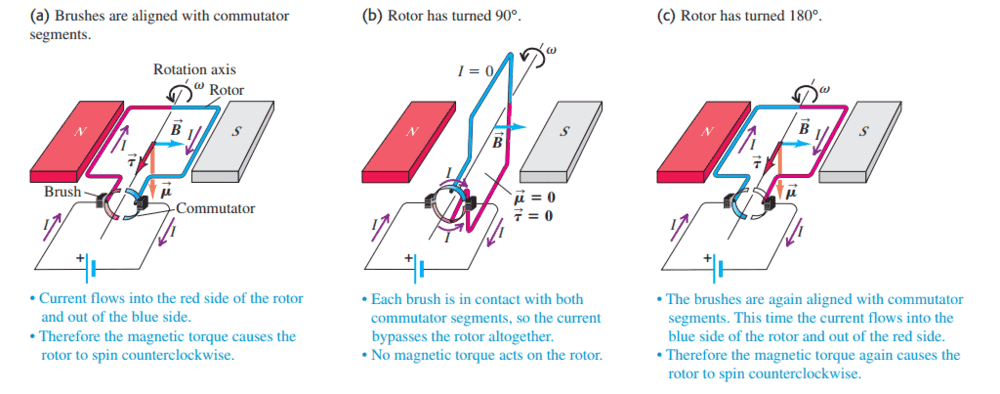Based on the above explanation i have drawn the torque curve. Can you please confirm if it is correct? In the initial position the torque is maximum and when it reaches the diagram 2 the torque is 0 and then it is maximum.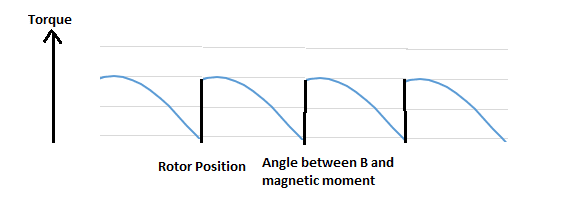Welcome to PF.
Is this homework?

The motor is symmetrical, so the torque curve should be symmetrical.
The commutator reverses the current flow at the point of minimum torque.
You need to label your x-axis with angle, and specify zero on the y-axis.

Welcome to PF.
Is this homework?
No. This is only for my understanding purpose and analysis.

The motor is symmetrical, so the torque curve should be symmetrical.
The commutator reverses the current flow at the point of minimum torque.
You need to label your x-axis with angle, and specify zero on the y-axis.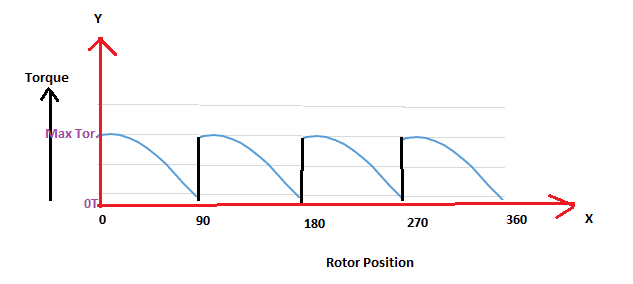Can you please confirm if the waveform is correct. I have few other clarifications once i understand this.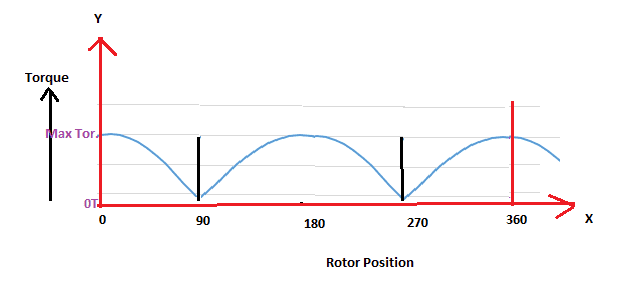•Physicslearner500039
Thank you now understand my mistake. Now one other clarification is
Another drawback of the simple design in is that the magnitude of the torque rises and falls as the rotor spins. This can be remedied by having the rotor include several independent coils of wire oriented at different angles. To confirm the construction i have drawn as an example two rotor coils. Let us say the loop1 is at 0 position and the loop2 at 30degrees wrt B field. Then the component of the torque which is perpendicular to B field will contribute. So, at rotor position 0 deg. The net torque will be T + T/2 = 3T/2. And at the rotor position of 30deg the torque is again 3T/2. I mean the net Torque will remain constant. If my approach is correct i want to go in details and increase the number of loops and do the calculations for each degree. Is my approach correct?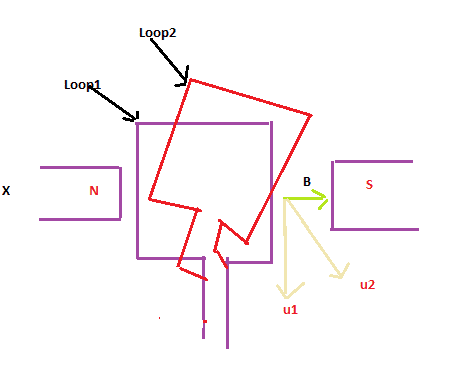Yes.
You will have many bars on the commutator, and many coils in armature slots, connected to bars on the commutator.
Notice that the brushes can contact several commutator bars at one time, so multiple coils near 90° are operating in parallel.
The two basic ways of connection are “wave” and “lap” winding.
https://en.wikipedia.org/wiki/Armature_(electrical)#Winding_circuits

Yes.
You will have many bars on the commutator, and many coils in armature slots, connected to bars on the commutator.
Notice that the brushes can contact several commutator bars at one time, so multiple coils near 90° are operating in parallel.
The two basic ways of connection are “wave” and “lap” winding.
https://en.wikipedia.org/wiki/Armature_(electrical)#Winding_circuits
Thank you for sharing. I have few other clarifications hope you can support me.
Q1. I am trying to correlate the theory with the practical diagram shared in the same book, theory it is ok but when it comes to the practical orientation seems to be difficult to interpret, so i have done an attempt to see if i understand correctly.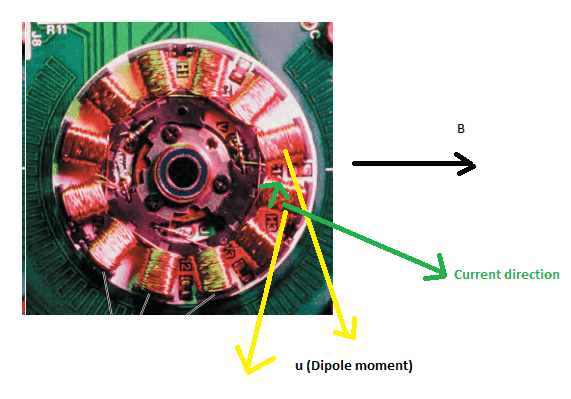In the diagram i cannot find the permanent magnets, so i am assuming the B field towards right, the current direction I am assuming as shown. Are all the directions i have shown are correct? The net torque because of all the coils will be the vector sum of independent coils and makes the rotor run in the anti-clockwise direction.
Q2. There is another way of explanation where because of the current on the rotors there is North and South poles induced on the coils and they try to align with the B field North and South. Can i apply the concept here? How to correlate the two concepts?

That looks like a brushless three phase synchronous motor with a tacho track on the PCB. The magnetic armature, disk and spindle has been removed. The disk would have covered the coils and had a rim with permanent magnets. Are you sure it is a real motor and not just techno-art?
What is the book you refer to? Title, author and year?

This is the explanation about the diagram
"This motor from a computer disk drive has 12 current-carrying coils. They interact with permanent magnets on the turntable (not shown) to make the turntable rotate. (This design is the reverse of the design in Fig. 27.39, in which the permanent magnets are stationary and the coil rotates.) Because there are multiple coils,the magnetic torque is very nearly constant and the turntable spins at a very constant rate."
University Physics, Young and Freedman, 13 edition.

The analysis of that motor takes you away from DC motors and into the field of three-phase synchronous motors.

Notice the middle of the motor is a metal plate and that each coil is wound on a radial extension of that plate. The magnetic poles are then the curved bar at the outer end of each coil. The voltages supplied to each group of three coils are separated by 120°. The next three coils receive the same signals and so on, 4 times around the 12 coil field winding.

There will be 8 PM poles on the rotating armature, that is 4 north poles, alternated with 4 south poles. Each group of three coils is moving a N and a S pole around the circumference. It takes 4 full cycles of the three phase signal to rotate the PM armature once.

The analysis of that motor takes you away from DC motors and into the field of three-phase synchronous motors.
I have some knowledge about 3 phase synchronous motors, but as usual i have lot of questions, since i find there is no single configuration possible.
Notice the middle of the motor is a metal plate and that each coil is wound on a radial extension of that plate. The magnetic poles are then the curved bar at the outer end of each coil. The voltages supplied to each group of three coils are separated by 120°. The next three coils receive the same signals and so on, 4 times around the 12 coil field winding.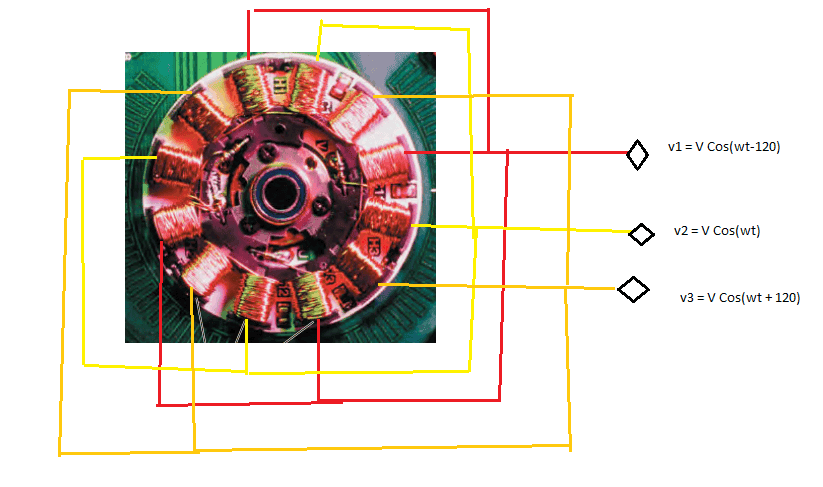I hope i have drawn correctly. Can you please confirm. I need more time to analyze the rotor connections you have explained. I try to draw that as well and want to confirm how the motor runs. Thanks for supporting.

Yes, that is the right idea.
Notice how the radial 8 pole field circulates, and drags the PM armature around with it.

I tried hard but could not completely understand the rotation, the picture as per my understanding
There will be 8 PM poles on the rotating armature, that is 4 north poles, alternated with 4 south poles.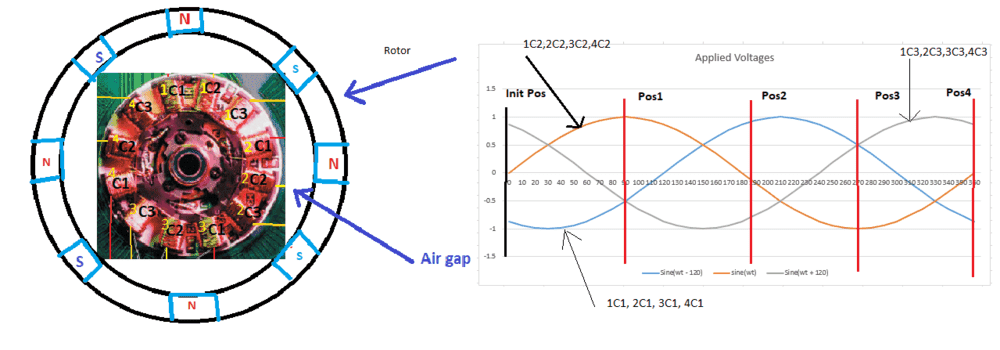I assume that the sets of stator coils are
a. 1C1, 2C2, 3C3 -> Set1
b. 1C2, 2C3, 4C1 -> Set2
c. 1C3, 3C1, 4C2 -> Set3
d. 2C1, 3C2, 4C3 -> Set4
the 3 phase sine voltages are applied with 120 Deg apart.
It takes 4 full cycles of the three phase signal to rotate the PM armature once.
I really want to understand this, why it takes 4 full cycles to rotate once. I have drawn here 1 full cycle, if i understand this, then i extend it for 4 cycles.
Because of the 4 sets (Set1, Set2, Set3, Set4) and since I applied the voltage / current wave-forms with 120 Degree apart I get 4 North and 4 South Poles, because of the 4 magnetic fields ##\vec B## caused by the waveforms. But i am confused with the position of the 4N and 4S poles, how to find this out. If I can do for the Initial position that is ##\omega t = 0## then I can extend for the complete 1 cycle.
Initial Position:
Step1: The phase voltages are applied with the values as shown, I switch to currents i think that is the standard. For a single set of coils 120 Degrees apart and when ##\omega t = 0 ## the net magnetic field is given by ##B_{net} = B_1 + B_2 + B_3 = 1.5B_M\angle{-90}##. Here also I do not know how the North and South poles align? Can you please clarify all my doubts?

Another explanation.

The video was helpful in understanding but few clarifications will help me to understand more clearly, I am not sure if they are mistakes in videos itself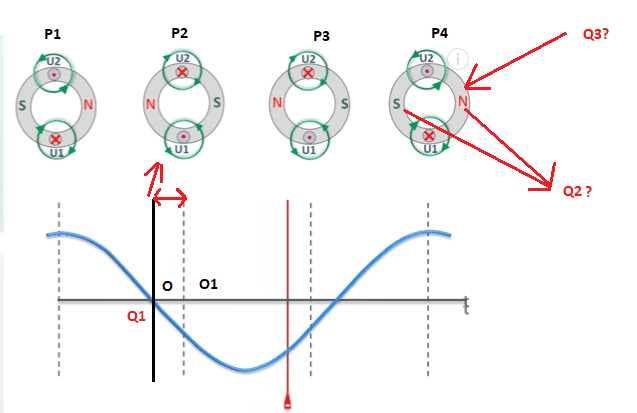Q1. I know that in P1, in U1 the magnetic field is going into the page and U2 is going into the page and they represent the magnetic field. I am only confused the graph represents the current and when the current goes into the negative region at "O", the magnetic field direction changes in U1 and U2 only at "O1". What is the reason, I am very confused?

Q2. For this I see the "S" south pole and "N" North pole appearing at a distance 45Deg and particularly in that order in P4 (in other positions as well). What is the logic behind this? My understanding is something like this.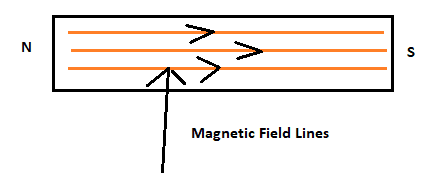The North and South pole are created based on the current direction for an electromagnet.

Q3. Very basic question what is that gray circles indicate actually?

in U1 the magnetic field is going into the page and U2 is going into the page and they represent the magnetic field.
After going through some of the other information, I realized it is the current not the magnetic field, and U2 is coming out of the page.

•Baluncore
Q2. For this I see the "S" south pole and "N" North pole appearing at a distance 45Deg and particularly in that order in P4 (in other positions as well). What is the logic behind this?
The example is a two pole, 3 phase rotating field. When one phase coil has maximum positive current the two other phase coils will have partial negative currents. Now select each of the six areas inside the stator and sum the fields due to the current in each of the three phase coils. As time passes the area with maximum North field will move around to the next area.

Your 45° does not come into it. The two magnetic poles are 180° apart, and separated from the peak windings by 90°. The three phase fields are 120° apart. The polarity of the six field areas changes every 60° phase.

Q3. Very basic question what is that gray circles indicate actually?
I believe the grey circle probably symbolises the magnetic stator material. The stator coil lies in slots on the inside surface of that magnetic material.

U1 and U2 are the same current in the same coil, flowing up one side and down the other side of the coil. Notice how the two circular magnetic fields due to the U1 and U2 currents reinforce.